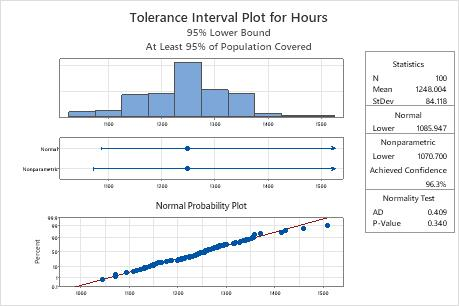# Example of Tolerance Intervals (Normal Distribution)

A quality engineer wants to assess the life span of the light bulbs that a company manufactures. The engineer collects a random sample of 100 bulbs and records the burn times to failure. The engineer wants to calculate the lower tolerance bound (which is the burn time that at least 95% of all light bulbs exceed).

1. Open the sample data, LightBulbBurnTime.MTW.
2. Choose Stat > Quality Tools > Tolerance Intervals (Normal Distribution).
3. From the drop-down list, select One or more samples, each in a column, then enter Hours.
4. Click Options.
5. From Tolerance interval, select Lower bound.
6. Click OK in each dialog box.

## Interpret the results

The normal probability plot shows that the plotted points form an approximate straight line, which indicates that the data follow a normal distribution. Also, the p-value for the normality test is 0.340, which is greater than the significance level (α = 0.05). Thus, there is not enough evidence to conclude that the data do not follow a normal distribution. The engineer can use the normal method results.

The normal lower bound is 1085.947, so the engineer is 95% confident that at least 95% of all the light bulbs exceed approximately 1086 hours of burn time. For all the light bulbs, the mean burn time is approximately 1248 hours and the standard deviation is approximately 84.1.By using this site you agree to the use of cookies for analytics and personalized content.  Read our policy